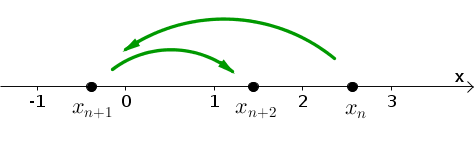# Math Insight

### Image: Two steps of function iteration on the number lineTwo iterations of a function of one variable leads to two jumps on the number line. If we start at the point $x_{n}$ on the number line, applying a function $f$ leads to the point $x_{n+1}=f(x_{n})$ and applying the function a second time leads to $x_{n+2}=f(x_{n+1})=f(f(x_n))$.

Image file: function_iteration_two_steps_number_line.png

Source image file: function_iteration_two_steps_number_line.ggb
Source image type: Geogebra